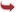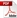International Journal of Scientific and Research Publications

#### IJSRP, Volume 6, Issue 11, November 2016 Edition [ISSN 2250-3153]Root Mean Square Derivative - Based Closed Newton Cotes Quadrature
T. Ramachandran, D.Udayakumar and R.Parimala
Abstract: In this paper, a set of Root mean square derivative - based closed Newton Cotes quadrature formula (RMSDCNC) is introduced in which the derivative value is included in addition to the existing closed Newton Cotes quadrature (CNC) formula for the calculation of a definite integral in the inetrval [a, b]. These derivative value is measured by using the root mean square value. The proposed formula yields improved precision than the existing formula. The error terms are likewise obtained by utilizing the method based on the precision of a Quadrature formula. Lastly, numerical examples are discussed to show the accuracy of the proposed rule.
[VIEW FULL PAPER][DOWNLOAD]

Reference this Research Paper (copy & paste below code):

T. Ramachandran, D.Udayakumar and R.Parimala (2018); Root Mean Square Derivative - Based Closed Newton Cotes Quadrature; Int J Sci Res Publ 6(11) (ISSN: 2250-3153). http://www.ijsrp.org/research-paper-1116.php?rp=P595948